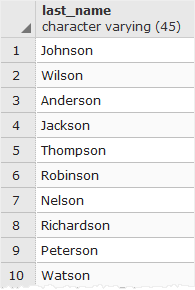# PostgreSQL Tutorial: RIGHT Function: Get Last N characters in a String

September 19, 2023

The PostgreSQL `RIGHT()` function returns the last `n` characters in a string.

## Syntax

The following shows the syntax of the PostgreSQL `RIGHT()` function:

``````RIGHT(string, n)
``````

## Arguments

The PostgreSQL `RIGHT()` function requires two arguments:

1) `string`

is a string from which a number of the rightmost characters returned.

2) `n`

is a positive integer which specifies the number of the rightmost characters in the string should be returned.

If `n` is negative, the `RIGHT()` function returns all characters in the string but first `|n|` (absolute) characters.

## Return value

The PostgreSQL `RIGHT()` function returns the last `n` characters in a string.

## Examples

Let’s look at some examples of using the PostgreSQL `RIGHT()` function.

The following statement gets the last character in the string `'XYZ'`:

``````SELECT RIGHT('XYZ', 1);
``````

Here is the result:

`````` right
-------
Z
(1 row)
``````

To get the last two characters, you pass the value `2` as the second argument as follows:

``````SELECT RIGHT('XYZ', 2);
``````

And the result is:

`````` right
-------
YZ
(1 row)
``````

The following statement illustrates how to use a negative integer as the second argument:

``````SELECT RIGHT('XYZ', - 1);
``````

In this example, the `RIGHT()` function returns all character except for the first character.

`````` right
-------
YZ
(1 row)
``````

See the following `customer` table in the sample database:The following statement uses the `RIGHT()` function in `WHERE` clause to get all customers whose last names ended with `'son'`:

``````SELECT last_name
FROM customer
WHERE RIGHT(last_name, 3) = 'son';
``````

The following picture illustrates the result:## Remarks

If you want to return the `n` first characters, please check the `LEFT()` function for more detailed information.

In this tutorial, you’ve learned how to use the PostgreSQL `RIGHT()` function to get the n rightmost characters in a string.